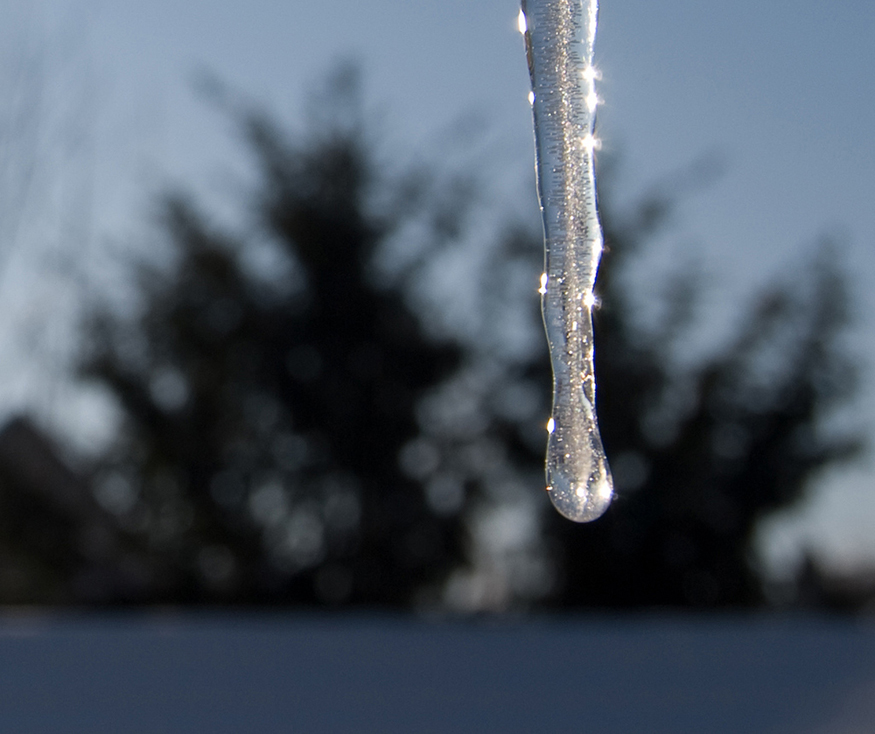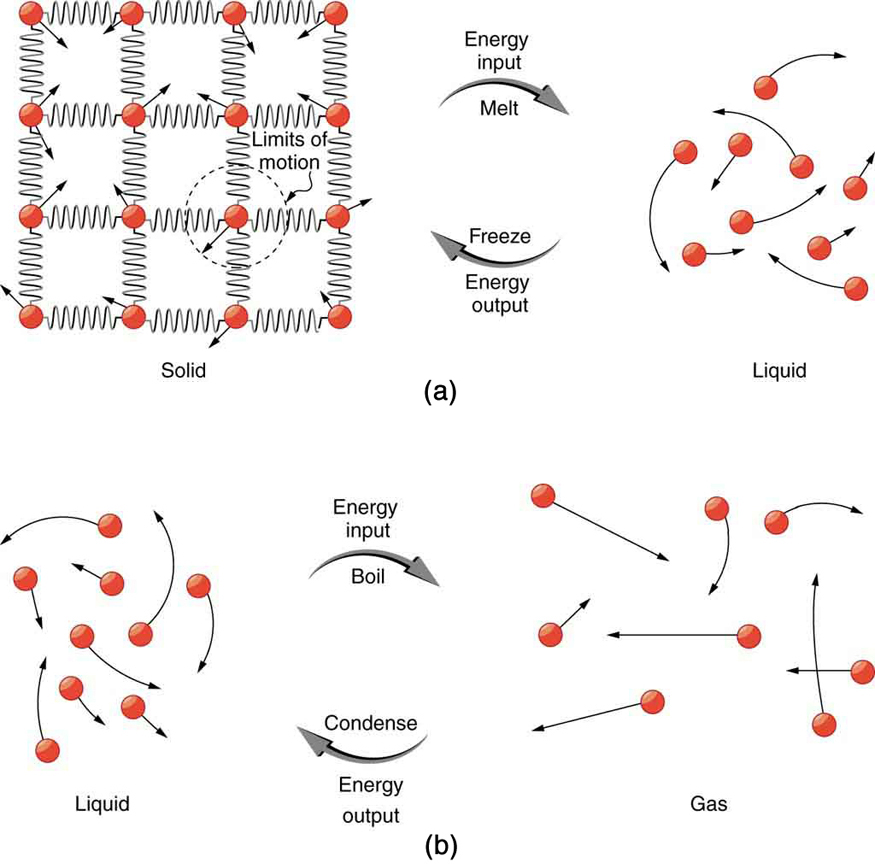# 14.3 Phase change and latent heat

 Page 1 / 9
• Examine heat transfer.
• Calculate final temperature from heat transfer.

So far we have discussed temperature change due to heat transfer. No temperature change occurs from heat transfer if ice melts and becomes liquid water (i.e., during a phase change). For example, consider water dripping from icicles melting on a roof warmed by the Sun. Conversely, water freezes in an ice tray cooled by lower-temperature surroundings.Heat from the air transfers to the ice causing it to melt. (credit: Mike Brand)

Energy is required to melt a solid because the cohesive bonds between the molecules in the solid must be broken apart such that, in the liquid, the molecules can move around at comparable kinetic energies; thus, there is no rise in temperature. Similarly, energy is needed to vaporize a liquid, because molecules in a liquid interact with each other via attractive forces. There is no temperature change until a phase change is complete. The temperature of a cup of soda initially at $0º\text{C}$ stays at $0º\text{C}$ until all the ice has melted. Conversely, energy is released during freezing and condensation, usually in the form of thermal energy. Work is done by cohesive forces when molecules are brought together. The corresponding energy must be given off (dissipated) to allow them to stay together [link] .

The energy involved in a phase change depends on two major factors: the number and strength of bonds or force pairs. The number of bonds is proportional to the number of molecules and thus to the mass of the sample. The strength of forces depends on the type of molecules. The heat $Q$ required to change the phase of a sample of mass $m$ is given by

where the latent heat of fusion, ${L}_{\text{f}}$ , and latent heat of vaporization, ${L}_{\text{v}}$ , are material constants that are determined experimentally. See ( [link] ).(a) Energy is required to partially overcome the attractive forces between molecules in a solid to form a liquid. That same energy must be removed for freezing to take place. (b) Molecules are separated by large distances when going from liquid to vapor, requiring significant energy to overcome molecular attraction. The same energy must be removed for condensation to take place. There is no temperature change until a phase change is complete.

Latent heat is measured in units of J/kg. Both ${L}_{\text{f}}$ and ${L}_{\text{v}}$ depend on the substance, particularly on the strength of its molecular forces as noted earlier. ${L}_{\text{f}}$ and ${L}_{\text{v}}$ are collectively called latent heat coefficients . They are latent , or hidden, because in phase changes, energy enters or leaves a system without causing a temperature change in the system; so, in effect, the energy is hidden. [link] lists representative values of ${L}_{\text{f}}$ and ${L}_{\text{v}}$ , together with melting and boiling points.

The table shows that significant amounts of energy are involved in phase changes. Let us look, for example, at how much energy is needed to melt a kilogram of ice at $0º\text{C}$ to produce a kilogram of water at $0°\text{C}$ . Using the equation for a change in temperature and the value for water from [link] , we find that $Q={\text{mL}}_{\text{f}}=\left(1\text{.}0\phantom{\rule{0.25em}{0ex}}\text{kg}\right)\left(\text{334}\phantom{\rule{0.25em}{0ex}}\text{kJ/kg}\right)=\text{334}\phantom{\rule{0.25em}{0ex}}\text{kJ}$ is the energy to melt a kilogram of ice. This is a lot of energy as it represents the same amount of energy needed to raise the temperature of 1 kg of liquid water from $0º\text{C}$ to $\text{79}\text{.}8º\text{C}$ . Even more energy is required to vaporize water; it would take 2256 kJ to change 1 kg of liquid water at the normal boiling point ( $\text{100º}\text{C}$ at atmospheric pressure) to steam (water vapor). This example shows that the energy for a phase change is enormous compared to energy associated with temperature changes without a phase change.

#### Questions & Answers

How is the de Broglie wavelength of electrons related to the quantization of their orbits in atoms and molecules?
How do you convert 0.0045kgcmÂ³ to the si unit?
how many state of matter do we really have like I mean... is there any newly discovered state of matter?
I only know 5: •Solids •Liquids •Gases •Plasma •Bose-Einstein condensate
Thapelo
Alright Thank you
Falana
Which one is the Bose-Einstein
James
can you explain what plasma and the I her one you mentioned
Olatunde
u can say sun or stars are just the state of plasma
Mohit
but the are more than seven
Issa
what the meaning of continuum
What state of matter is fire
fire is not in any state of matter...fire is rather a form of energy produced from an oxidising reaction.
Xenda
Isn`t fire the plasma state of matter?
Walter
all this while I taught it was plasma
Victor
How can you define time?
Time can be defined as a continuous , dynamic , irreversible , unpredictable quantity .
Tanaya
unpredictable? but I can say after one o'clock its going to be two o'clock predictably!
Victor
what is the relativity of physics
How do you convert 0.0045kgcm³ to the si unit?
flint
What is the formula for motion
V=u+at V²=u²-2as
flint
S=ut+½at
flint
they are eqns of linear motion
King
S=Vt
Thapelo
v=u+at s=ut+at^\2 v^=u^+2as where ^=2
King
hi
hello
King
Explain dopplers effect
Not yet learnt
Bob
Explain motion with types
Bob
Acceleration is the change in velocity over time. Given this information, is acceleration a vector or a scalar quantity? Explain.
Scalar quantity Because acceleration has only magnitude
Bob
acleration is vectr quatity it is found in a spefied direction and it is product of displcemnt
bhat
its a scalar quantity
Paul
velocity is speed and direction. since velocity is a part of acceleration that makes acceleration a vector quantity. an example of this is centripetal acceleration. when you're moving in a circular patter at a constant speed, you are still accelerating because your direction is constantly changing.
Josh
acceleration is a vector quantity. As explained by Josh Thompson, even in circular motion, bodies undergoing circular motion only accelerate because on the constantly changing direction of their constant speed. also retardation and acceleration are differentiated by virtue of their direction in
fitzgerald
respect to prevailing force
fitzgerald
What is the difference between impulse and momentum?
Manyo
Momentum is the product of the mass of a body and the change in velocity of its motion. ie P=m(v-u)/t (SI unit is kgm/s). it is literally the impact of collision from a moving body. While Impulse is the product of momentum and time. I = Pt (SI unit is kgm) or it is literally the change in momentum
fitzgerald
Or I = m(v-u)
fitzgerald
Calculation of kinetic and potential energy
K.e=mv² P.e=mgh
Malia
K is actually 1/2 mv^2
Josh
what impulse is given to an a-particle of mass 6.7*10^-27 kg if it is ejected from a stationary nucleus at a speed of 3.2*10^-6ms²? what average force is needed if it is ejected in approximately 10^-8 s?
John
speed=velocity÷time velocity=speed×time=3.2×10^-6×10^-8=32×10^-14m/s impulse [I]=∆momentum[P]=mass×velocity=6.7×10^-27×32×10^-14=214.4×10^-41kg/ms force=impulse÷time=214.4×10^-41÷10^-8=214.4×10^-33N. dats how I solved it.if wrong pls correct me.
Melody
what is sound wave
sound wave is a mechanical longitudinal wave that transfers energy from one point to another
Ogor
its a longitudnal wave which is associted wth compresion nad rearfractions
bhat
what is power
it's also a capability to do something or act in a particular way.
Kayode
Newton laws of motion
Mike
power also known as the rate of ability to do work
Slim
power means capabilty to do work p=w/t its unit is watt or j/s it also represents how much work is done fr evry second
bhat
what does fluorine do?
strengthen and whiten teeth.
Gia
a simple pendulum make 50 oscillation in 1minute, what is it period of oscillation?
length of pendulm?
bhatByBy